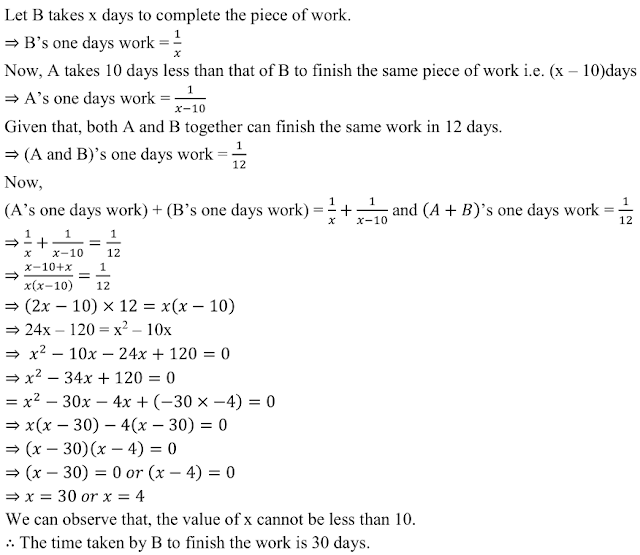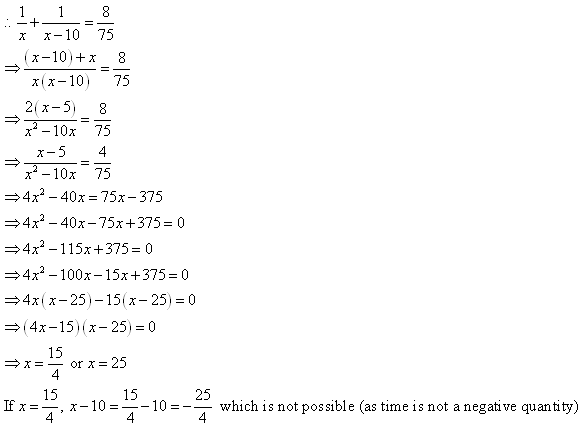## Chapter 8 Quadratic Equations R.D. Sharma Solutions for Class 10th Math Exercise 8.12

1. A takes 10 days less than the time taken by B to finish a piece of work. If both A and B together can finish the work in 12 days, find the time taken by B to finish the work.

Solution2. If two pipes function simultaneously, a reservoir will be filled in 12 hours. One pipe fills the reservoir 10 hours faster than the other. How many hours will the second pipe take to fill the reservoir?

Solution

Let the faster pipe fill the reservoir in x h.
Then, the  slower pipe the reservoir in (x + 10) h
In 1 hour the faster pipe fills the portion of the reservoir : 1/x
In 12 hour the faster pipe fills the portion of the reservoir : 12 ×  1/x  = 12/x
In 1 hour the slower pipe fills the portion of the reservoir : 1/(x + 10)
In 12 hour the faster pipe fills the portion of the reservoir : 12 × 1/(x + 10) = 12/(x +10)
A/q,
12/x + 12/(x +10) = 1
⇒ 12(1/x + 1/(x + 10) ) = 1
⇒ 1/x + 1/(x + 10) = 1/12
⇒ (x + 10 + x ) / [x(x + 10)] = 1/12
[By taking LCM]
⇒ 2x +10 /(x² + 10x) = 1/12
⇒ x² + 10x = 12(2x +10)
⇒ x² + 10x = 24x + 120
⇒ x² + 10x - 24x - 120 = 0
⇒ x² - 14x - 120 = 0
⇒ x² + 6x - 20x - 120 = 0
⇒ x(x + 6) - 20(x + 6) = 0
⇒ (x - 20) (x + 6) = 0
⇒ (x - 20) or  (x + 6) = 0
⇒ x = 20  or  x = - 6
Since, time cannot be negative, so x ≠ - 6
Therefore, x = 20
The faster pipe takes 20 hours to fill the reservoir
Hence, the slower pipe (second) takes (x + 20) = 30 hours to fill the reservoir.

3. Two water taps together can fill a tank in 9 (3/8) hours. The tap of larger diameter takes 10 hours less than the smaller one to fill the tank separately. Find the time in which each tap can separately fill the tank.

Solution

Let the tap with smaller diameter fills the tank alone in x hours.
Let the tap with larger diameter fills the tank alone in (x – 10) hours.
In 1 hour, the tap with smaller diameter can fill 1/x part of the tank.
In 1 hour, the tap with larger diameter can fill 1/(x – 10) part of the tank.
The tank is filled up in 75/8 hours.
Thus, in 1 hour the taps fill 8/75 part of the tank.If x = 25, then (x – 10) = 25 – 10 = 15.
Thus, the tap with smaller diameter fills the tank alone in 25 hours where as the larger diameter fills the tank alone in 15 hours.

4. Two pipes running together can fill a tank in 11(1/9) minutes. If one pipe takes 5 minutes more than the other to fill the tank separately, find the time in which each pipe would fill the tank separately.

Solution

Let one of the pipe take x minutes to then the slower pipe will take (x+5) minutes.
Time taken by both pipe to fill the tank = 100/9 minutes
Portion of tank filled by faster pipe in 1 minute = 1/x
Portion of tank filled by faster pipe in 1 minute = 1/(x+5)
Portion of tank filled by both pipe in one 1 minute = 1/(100/9) = 9/100
A/q,
1/x + 1/(x+5) = 9/100
⇒ (x+5 + x)/{x(x+5)} = 9/100
⇒ (2x+5)/(x²+5x) = 9/100
⇒ 200x + 500 = 9x² + 45x
⇒ 9x² + 45x - 200x - 500 = 0
⇒ 9x² - 155x - 500 = 0
⇒ 9x² - 180x + 25x  - 500 = 0
⇒ 9x (x - 20) + 25 (x - 20) = 0
⇒ (9x + 25) (x - 20) = 0
x = -25/9 and x = 20
But x can't be negative as it represents time.
Therefor, X = 20 minutes.
Time taken by faster pipe to fill the tank = 20 minutes.
Time taken by slower pipe to fill the tank = 20+5 = 25 minutes.

5. To fill a swimming pool two pipes are used. If the pipe of larger diameter used for 4 hours and the pipe of smaller diameter for 9 hours, only half of the pool can be filled. Find, how long it would take for each pipe to fill the pool separately, if the pipe of smaller diameter takes 10 hours more than this pipe of larger diameter to fill the pool ?

Solution